# Transient Modeling and Linear Analysis of a Cantilever Beam

This example shows how to obtain a finite element model of a beam and calculate the response when subjected to an impulse force. For this example, consider a steel cantilever beam subject to a point load at the tip. Building the finite element model requires a Partial Differential Equation Toolbox license.

The damping model is basic viscous damping distributed uniformly through the volume of the beam. The steel beam is deformed by applying an external load of 100 N at the tip of the beam and then released. This example does not use any additional loading, so the displacement of the beam decreases as a function of time due to the damping. This example follows the three-step workflow:

1. Transient analysis of the beam model.

2. Extract sparse finite element matrices and construct the second-order sparse state-space model.

3. Perform linear analysis on the sparse second-order model.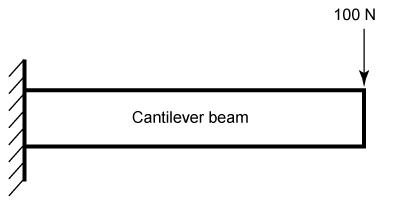### Transient Beam Model

Build a transient model and compute the impulse response.

First construct the beam and specify the Young's modulus, Poisson's ratio, and mass density of steel. Specify the tip of the beam using the `addVertex` property.

```gm = multicuboid(0.1,0.005,0.005); E = 210E9; nu = 0.3; rho = 7800; TipVertex = gm.addVertex('Coordinates',[0.05,0,0.005]); firstNF = 2639; Tfundamental = 2*pi/firstNF;```

Use `createpde` (Partial Differential Equation Toolbox) to construct the transient model and generate the mesh using the `generateMesh` (Partial Differential Equation Toolbox) command.

```transientModel = createpde('structural','transient-solid'); transientModel.Geometry = gm; msh = generateMesh(transientModel);```

Assign structural properties for the steel beam with the `structuralProperties` (Partial Differential Equation Toolbox) command and fix one end using `structuralBC` (Partial Differential Equation Toolbox).

```structuralProperties(transientModel,'YoungsModulus',E,'PoissonsRatio',nu,'MassDensity',rho); structuralBC(transientModel,'Face',5,'Constraint','fixed');```

Visualize the beam geometry using `pdegplot` (Partial Differential Equation Toolbox).

```pdegplot(transientModel,'VertexLabels','on'); title('Beam model')```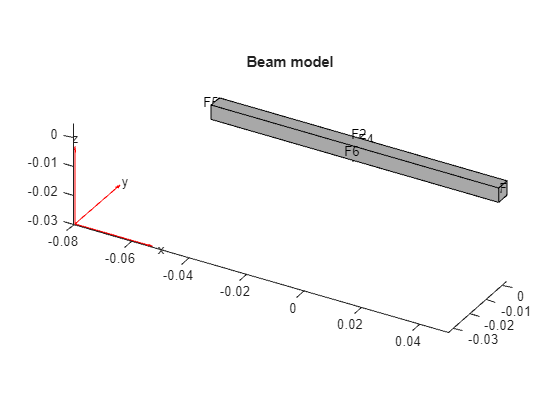Apply force on beam tip for 2% of fundamental period of oscillation (impulse) using `structuralBoundaryLoad` (Partial Differential Equation Toolbox).

```Te = 0.02*Tfundamental; structuralBoundaryLoad(transientModel,'Vertex',TipVertex,... 'Force',[0;0;-100],'EndTime',Te);```

Set initial conditions for the transient beam model using `structuralIC` (Partial Differential Equation Toolbox).

`structuralIC(transientModel,'Velocity',[0;0;0]);`

Compute the impulse response by solving the transient beam model.

```ncycles = 10; tsim = linspace(0,ncycles*Tfundamental,30*ncycles); R1 = solve(transientModel,tsim);```

Visualize the oscillations at the tip of the beam.

```plot(tsim,R1.Displacement.uz(TipVertex,:)) title('Vertical displacement of beam tip') legend('Transient model') xlabel('Time') ylabel('Displacement')```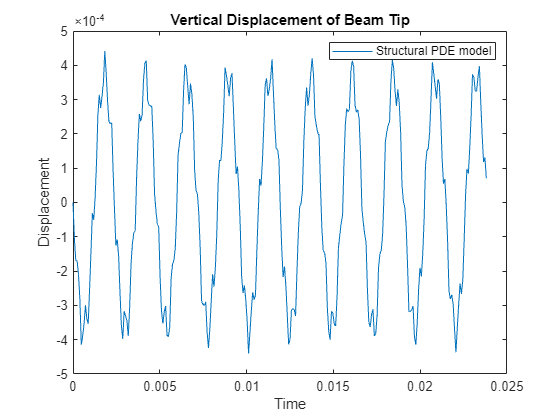The next step is to extract the sparse matrices from the finite element beam model.

### Sparse Model from the Transient Model

The first step to extract the `M`,`K`,`B`,`F` matrices from the finite element beam model to modulate the force to compute the `B` matrix. Use `assembleFEMatrices` (Partial Differential Equation Toolbox) to extract the sparse matrices for the second-order beam model.

```F1 = -100; structuralBoundaryLoad(transientModel,'Vertex',TipVertex,'Force',[0;0;F1]); state.time = 0; data1 = assembleFEMatrices(transientModel,'nullspace',state); F2 = 100; structuralBoundaryLoad(transientModel,'Vertex',TipVertex,'Force',[0;0;F2]); data2 = assembleFEMatrices(transientModel,'nullspace',state);```

The resultant model is defined by the following set of equations:

`$\begin{array}{l}M\underset{}{\overset{¨}{q}}+Kq=B*force\\ y=Fq\end{array}$`

```M = data1.M; K = data1.Kc;```

The `F` matrix consists of x, y and z displacements at the `TipVertex` node.

```N = size(msh.Nodes,2); F = data1.B; F = F(TipVertex+[0 N 2*N],:);```

Map the `B` matrix relating the force to the input.

`B = (data2.Fc-data1.Fc)/(F2-F1);`

Obtain the sparse model of the beam using `mechss`.

`sys = mechss(M,[],K,B,F(3,:));`

Use `spy` to visualize the sparsity of the mechss model `sys`.

`spy(sys)`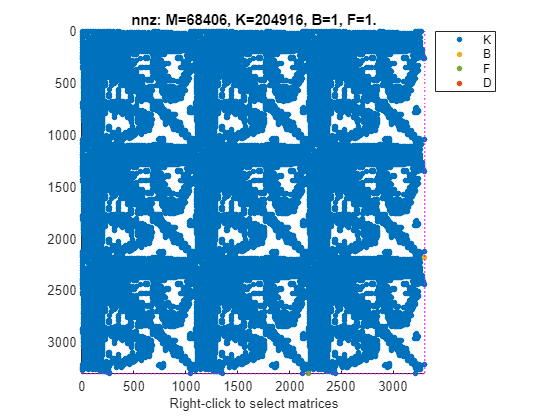### Linear Analysis

Enable parallel computing and choose `'tfbdf3'` as the DAE solver.

```sys.SolverOptions.UseParallel = true; sys.SolverOptions.DAESolver = 'trbdf3';```

Use `bode` to compute the frequency response of this model.

```w = logspace(2,6,1000); bode(sys,w), grid```
```using parallel (mechss) ```
`title('Frequency response from force to tip vertical displacement')`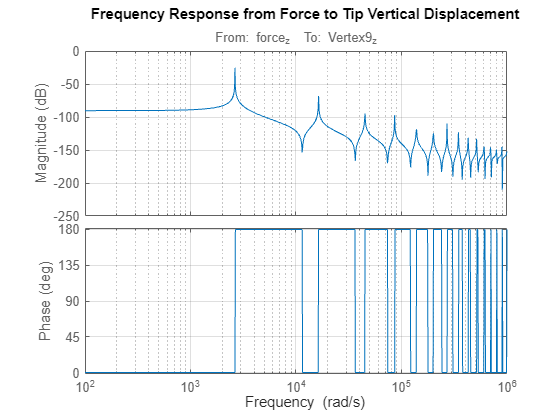Next use `lsim` to compare the impulse response with the approximations obtained from the transient beam model. To limit error due to linear interpolation of force between samples, use a step size of `Te/5`. The force applied at the beam tip is $0<=t<=\mathrm{Te}$.

```h = Te/5; t = 0:h:ncycles*Tfundamental; u = zeros(size(t)); u(t<=Te) = -100; y = lsim(sys,u,t); plot(t,y,tsim,R1.Displacement.uz(TipVertex,:)) title('Comparison of full-model simulated response with transient model approximations') legend('Simulated model','Transient model') xlabel('Time') ylabel('Displacement')```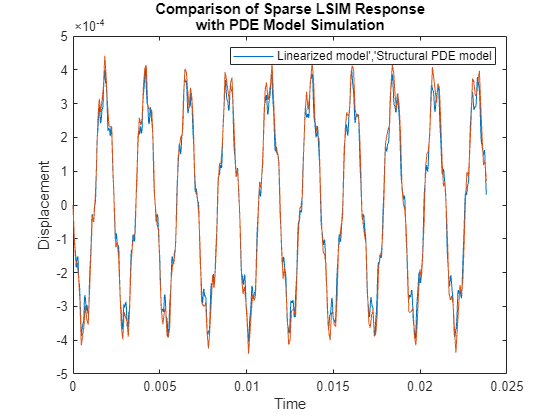The transient model approximations match well with the full-model simulation using the TRBDF3 DAE solver.